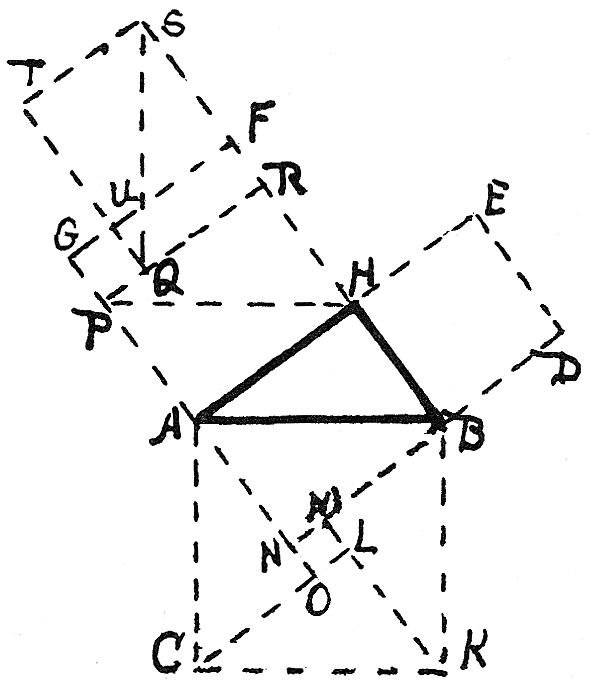#The Pythagorean Proposition: Geometric Proofs, Proof #32

Math Lair Home > Source Material > The Pythagorean Proposition > Geometric Proofs, Proof #32

### Thirty-TwoFig. 133

Extend GA making AD = AO.  Extend DB to N, draw CL and KM.  Extend BF to S making FS = HB, complete sq. SU, draw HP par. to AB, PR par. to AH and draw SQ.

Then, obvious, sq. AK = 4 tri. BAN + sq. NL
= rect. AR + rect. TR + sq. GQ = rect. AR + rect. QF
+ sq. GQ + (sq. TF = sq. ND) = sq. HG + sq. HD.  ∴ sq. upon AB = sq. upon BH + sq. upon AH.  ∴ h² = a² + b². Q.E.D.

a. This proof is credited to Miss E. A. Coolidge, a blind girl. See Journal of Education,
V. XXVIII, 1888, p. 17, 26th proof.

b. The reader will note that this proof em­ploys exactly the same dissection and arrangement as found in the solution by the Hindu mathematician, Bhaskara. See fig. 324, proof Two Hundred Twenty- Five.Geometric Proofs, proof #31
For more information on this work, see The Pythagorean Proposition by Elisha Scott Loomis.